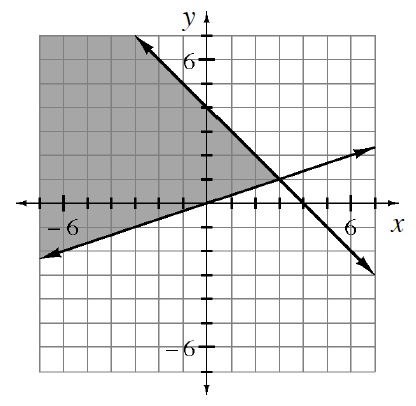### Home > CCA2 > Chapter Ch6 > Lesson 6.1.3 > Problem6-38

6-38.

Write the system of inequalities that would give the graph at right.

Find the y-intercepts and slopes of the lines by looking at the graph.

Determine the equations of the lines and then write the inequalities.

$y\le−x+4$